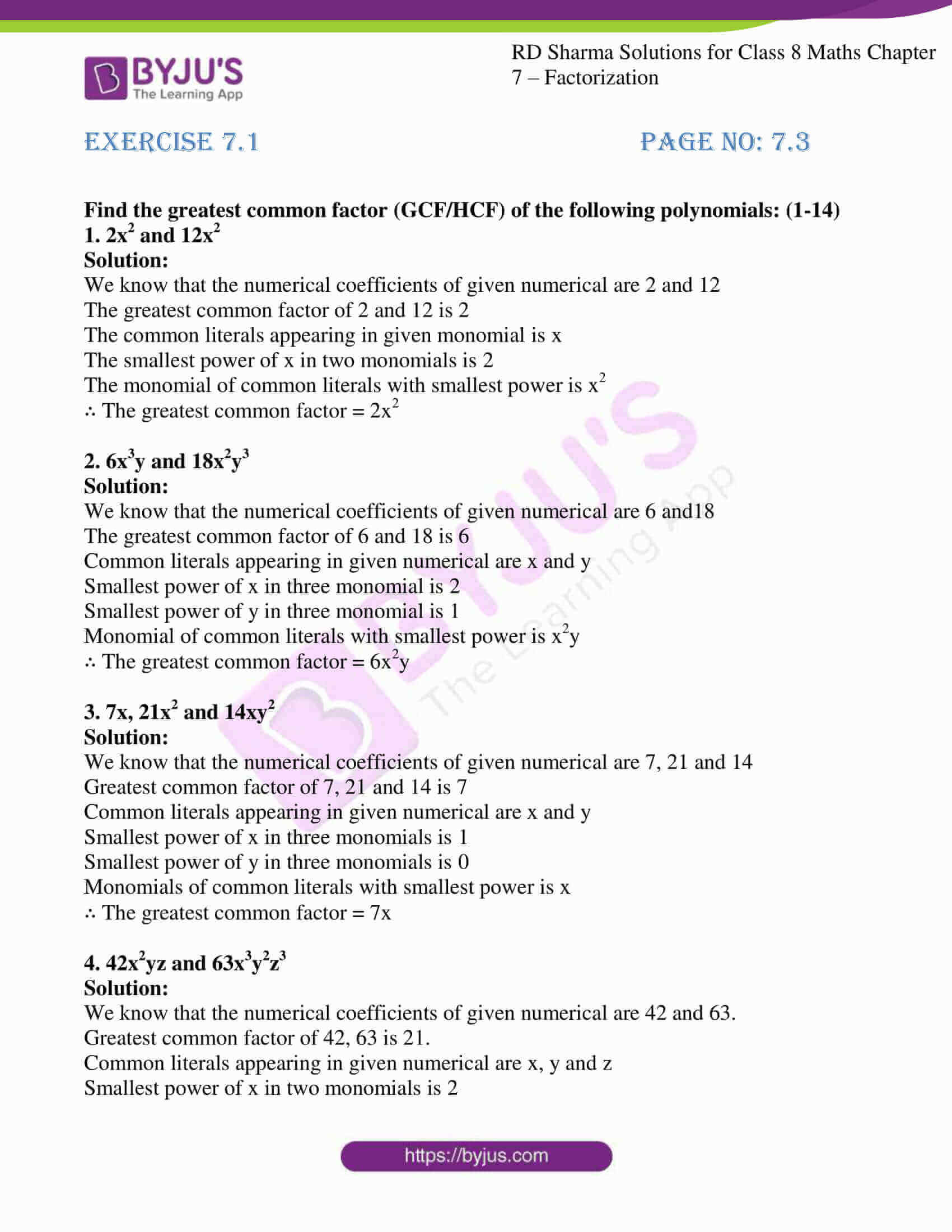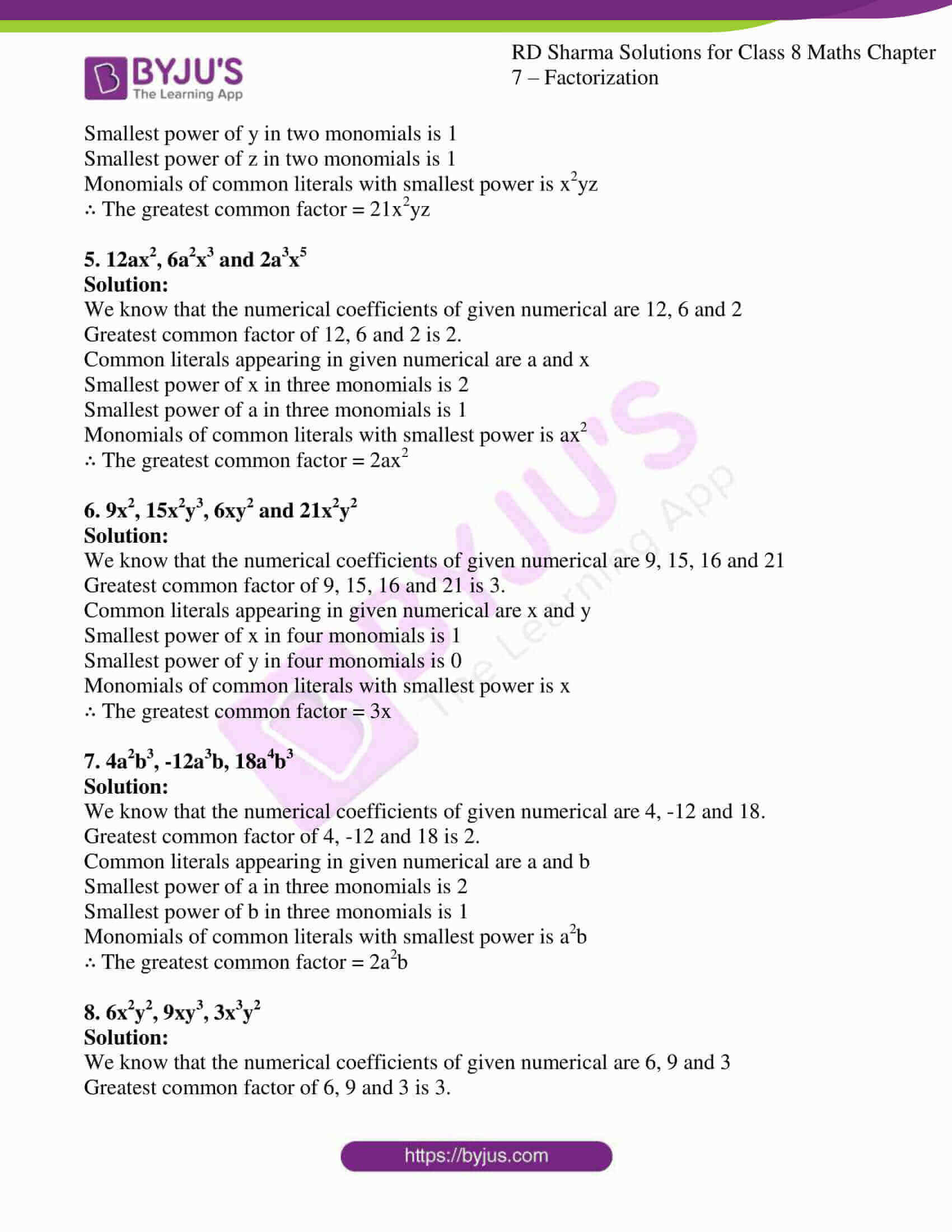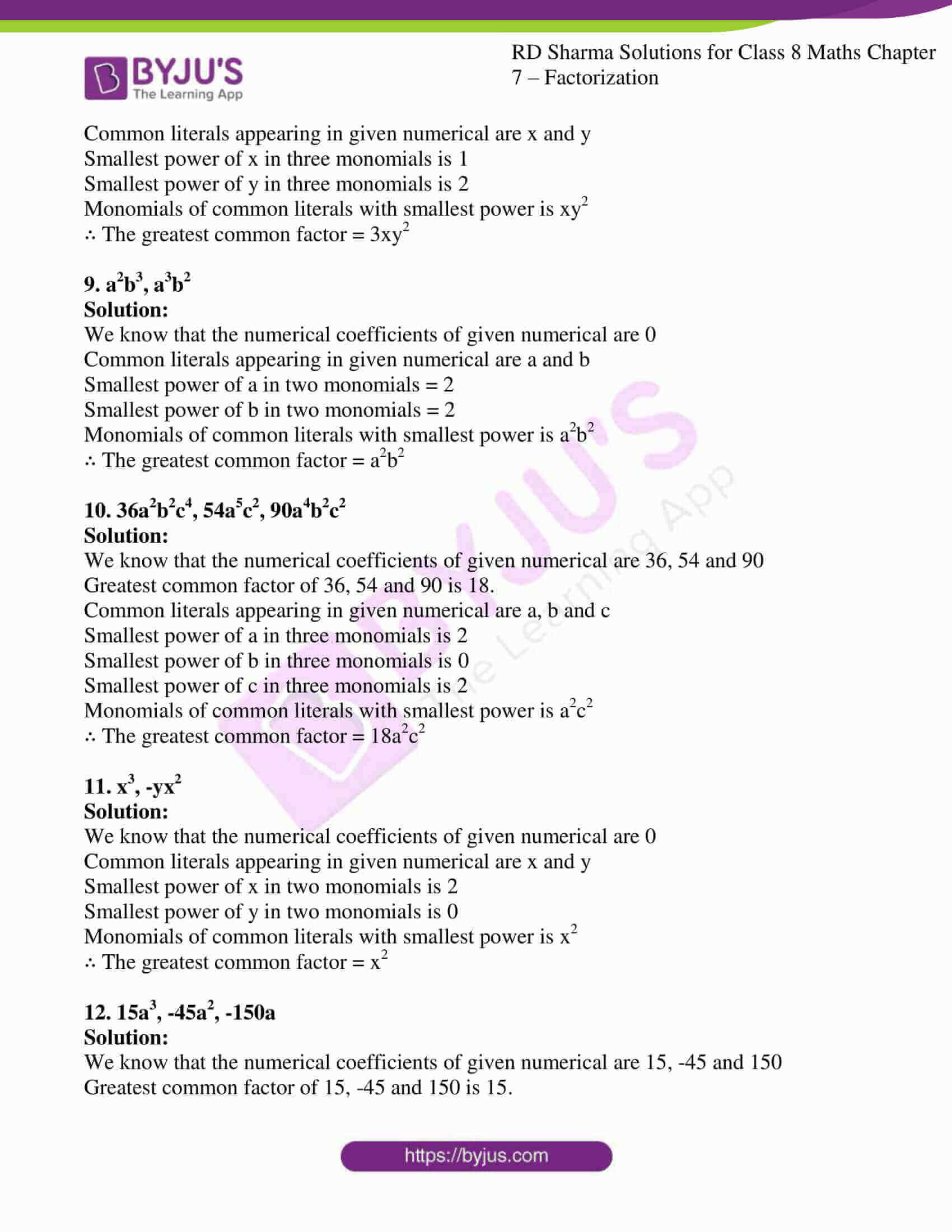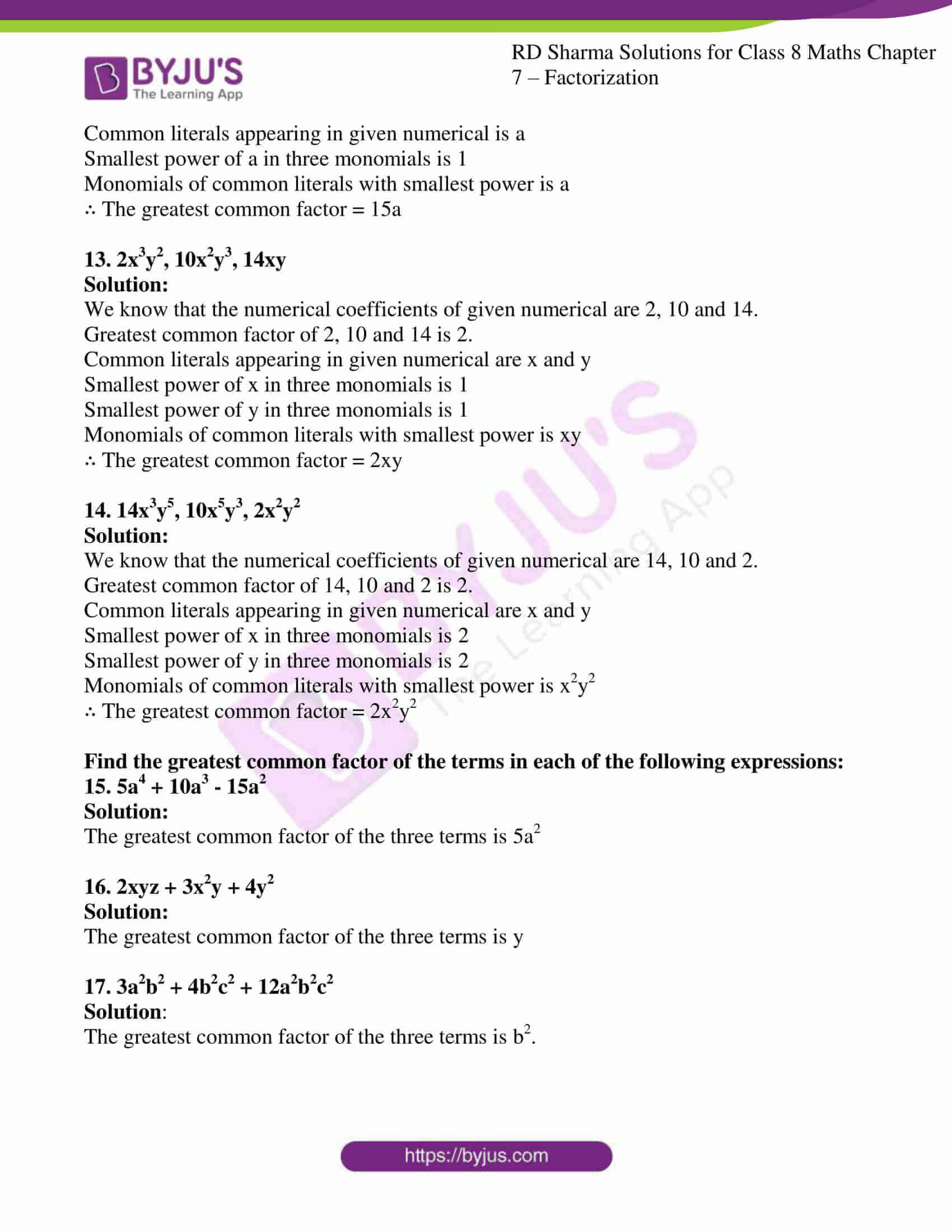# RD Sharma Solutions for Class 8 Maths Chapter - 7 Factorization Exercise 7.1

Our expert team has designed the solutions for RD Sharma Class 8 Maths Chapter 7 to help students prepare for their exams at ease. RD Sharma Solutions is one of the best reference material for CBSE students. Learners can download the pdf from the links provided below. Experts suggest students practice the solutions many numbers of times to yield good results in their exams. In Exercise 7.1 of Chapter 7 Factorization, we shall discuss the basic definitions for factors, factorization and we also find the common factors and greatest common factor of the monomial.

## Download the pdf of RD Sharma Solutions For Class 8 Maths Exercise 7.1 Chapter 7 Factorization### Access Answers to RD Sharma Solutions for Class 8 Maths Exercise 7.1 Chapter 7 Factorization

#### EXERCISE 7.1 PAGE NO: 7.3

Find the greatest common factor (GCF/HCF) of the following polynomials: (1-14)

1. 2x2 and 12x2

Solution:

We know that the numerical coefficients of given numerical are 2 and 12

The greatest common factor of 2 and 12 is 2

The common literals appearing in given monomial is x

The smallest power of x in two monomials is 2

The monomial of common literals with smallest power is x2

∴ The greatest common factor = 2x2

2. 6x3y and 18x2y3

Solution:

We know that the numerical coefficients of given numerical are 6 and18

The greatest common factor of 6 and 18 is 6

Common literals appearing in given numerical are x and y

Smallest power of x in three monomial is 2

Smallest power of y in three monomial is 1

Monomial of common literals with smallest power is x2y

∴ The greatest common factor = 6x2y

3. 7x, 21x2 and 14xy2

Solution:

We know that the numerical coefficients of given numerical are 7, 21 and 14

Greatest common factor of 7, 21 and 14 is 7

Common literals appearing in given numerical are x and y

Smallest power of x in three monomials is 1

Smallest power of y in three monomials is 0

Monomials of common literals with smallest power is x

∴ The greatest common factor = 7x

4. 42x2yz and 63x3y2z3

Solution:

We know that the numerical coefficients of given numerical are 42 and 63.

Greatest common factor of 42, 63 is 21.

Common literals appearing in given numerical are x, y and z

Smallest power of x in two monomials is 2

Smallest power of y in two monomials is 1

Smallest power of z in two monomials is 1

Monomials of common literals with smallest power is x2yz

∴ The greatest common factor = 21x2yz

5. 12ax2, 6a2x3 and 2a3x5

Solution:

We know that the numerical coefficients of given numerical are 12, 6 and 2

Greatest common factor of 12, 6 and 2 is 2.

Common literals appearing in given numerical are a and x

Smallest power of x in three monomials is 2

Smallest power of a in three monomials is 1

Monomials of common literals with smallest power is ax2

∴ The greatest common factor = 2ax2

6. 9x2, 15x2y3, 6xy2 and 21x2y2

Solution:

We know that the numerical coefficients of given numerical are 9, 15, 16 and 21

Greatest common factor of 9, 15, 16 and 21 is 3.

Common literals appearing in given numerical are x and y

Smallest power of x in four monomials is 1

Smallest power of y in four monomials is 0

Monomials of common literals with smallest power is x

∴ The greatest common factor = 3x

7. 4a2b3, -12a3b, 18a4b3

Solution:

We know that the numerical coefficients of given numerical are 4, -12 and 18.

Greatest common factor of 4, -12 and 18 is 2.

Common literals appearing in given numerical are a and b

Smallest power of a in three monomials is 2

Smallest power of b in three monomials is 1

Monomials of common literals with smallest power is a2b

∴ The greatest common factor = 2a2b

8. 6x2y2, 9xy3, 3x3y2

Solution:

We know that the numerical coefficients of given numerical are 6, 9 and 3

Greatest common factor of 6, 9 and 3 is 3.

Common literals appearing in given numerical are x and y

Smallest power of x in three monomials is 1

Smallest power of y in three monomials is 2

Monomials of common literals with smallest power is xy2

∴ The greatest common factor = 3xy2

9. a2b3, a3b2

Solution:

We know that the numerical coefficients of given numerical are 0

Common literals appearing in given numerical are a and b

Smallest power of a in two monomials = 2

Smallest power of b in two monomials = 2

Monomials of common literals with smallest power is a2b2

∴ The greatest common factor = a2b2

10. 36a2b2c4, 54a5c2, 90a4b2c2

Solution:

We know that the numerical coefficients of given numerical are 36, 54 and 90

Greatest common factor of 36, 54 and 90 is 18.

Common literals appearing in given numerical are a, b and c

Smallest power of a in three monomials is 2

Smallest power of b in three monomials is 0

Smallest power of c in three monomials is 2

Monomials of common literals with smallest power is a2c2

∴ The greatest common factor = 18a2c2

11. x3, -yx2

Solution:

We know that the numerical coefficients of given numerical are 0

Common literals appearing in given numerical are x and y

Smallest power of x in two monomials is 2

Smallest power of y in two monomials is 0

Monomials of common literals with smallest power is x2

∴ The greatest common factor = x2

12. 15a3, -45a2, -150a

Solution:

We know that the numerical coefficients of given numerical are 15, -45 and 150

Greatest common factor of 15, -45 and 150 is 15.

Common literals appearing in given numerical is a

Smallest power of a in three monomials is 1

Monomials of common literals with smallest power is a

∴ The greatest common factor = 15a

13. 2x3y2, 10x2y3, 14xy

Solution:

We know that the numerical coefficients of given numerical are 2, 10 and 14.

Greatest common factor of 2, 10 and 14 is 2.

Common literals appearing in given numerical are x and y

Smallest power of x in three monomials is 1

Smallest power of y in three monomials is 1

Monomials of common literals with smallest power is xy

∴ The greatest common factor = 2xy

14. 14x3y5, 10x5y3, 2x2y2

Solution:

We know that the numerical coefficients of given numerical are 14, 10 and 2.

Greatest common factor of 14, 10 and 2 is 2.

Common literals appearing in given numerical are x and y

Smallest power of x in three monomials is 2

Smallest power of y in three monomials is 2

Monomials of common literals with smallest power is x2y2

∴ The greatest common factor = 2x2y2

Find the greatest common factor of the terms in each of the following expressions:

15. 5a4 + 10a3 – 15a2

Solution:

The greatest common factor of the three terms is 5a2

16. 2xyz + 3x2y + 4y2

Solution:

The greatest common factor of the three terms is y

17. 3a2b2 + 4b2c2 + 12a2b2c2

Solution:

The greatest common factor of the three terms is b2.